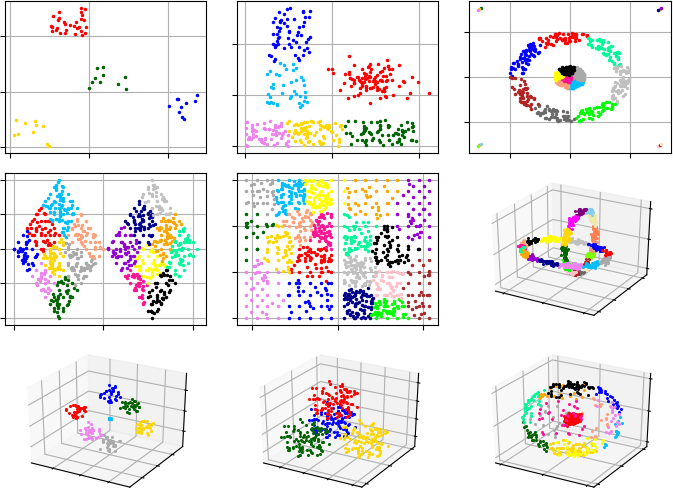pyclustering  0.10.1 pyclustring is a Python, C++ data mining library.
pyclustering.cluster.gmeans.gmeans Class Reference

Class implements G-Means clustering algorithm. More...

## Public Member Functions

def __init__ (self, data, k_init=1, ccore=True, **kwargs)
Initializes G-Means algorithm. More...

def process (self)
Performs cluster analysis in line with rules of G-Means algorithm. More...

def predict (self, points)
Calculates the closest cluster to each point. More...

def get_clusters (self)
Returns list of allocated clusters, each cluster contains indexes of objects in list of data. More...

def get_centers (self)
Returns list of centers of allocated clusters. More...

def get_total_wce (self)
Returns sum of metric errors that depends on metric that was used for clustering (by default SSE - Sum of Squared Errors). More...

def get_cluster_encoding (self)
Returns clustering result representation type that indicate how clusters are encoded. More...

## Detailed Description

Class implements G-Means clustering algorithm.

The G-means algorithm starts with a small number of centers, and grows the number of centers. Each iteration of the G-Means algorithm splits into two those centers whose data appear not to come from a Gaussian distribution. G-means repeatedly makes decisions based on a statistical test for the data assigned to each center.

Implementation based on the paper .G-Means clustering results on most common data-sets.

Example #1. In this example, G-Means starts analysis from single cluster.

from pyclustering.cluster import cluster_visualizer
from pyclustering.cluster.gmeans import gmeans
from pyclustering.samples.definitions import FCPS_SAMPLES
# Read sample 'Lsun' from file.
# Create instance of G-Means algorithm. By default the algorithm starts search from a single cluster.
gmeans_instance = gmeans(sample).process()
# Extract clustering results: clusters and their centers
clusters = gmeans_instance.get_clusters()
centers = gmeans_instance.get_centers()
# Print total sum of metric errors
print("Total WCE:", gmeans_instance.get_total_wce())
# Visualize clustering results
visualizer = cluster_visualizer()
visualizer.append_clusters(clusters, sample)
visualizer.show()

Example #2. Sometimes G-Means might find local optimum. repeat value can be used to increase probability to find global optimum. Argument repeat defines how many times K-Means clustering with K-Means++ initialization should be run in order to find optimal clusters.

# Read sample 'Tetra' from file.
# Create instance of G-Means algorithm. By default algorithm start search from single cluster.
gmeans_instance = gmeans(sample, repeat=10).process()
# Extract clustering results: clusters and their centers
clusters = gmeans_instance.get_clusters()
# Visualize clustering results
visualizer = cluster_visualizer()
visualizer.append_clusters(clusters, sample)
visualizer.show()

In case of requirement to have labels instead of default representation of clustering results CLUSTER_INDEX_LIST_SEPARATION:

from pyclustering.cluster.gmeans import gmeans
from pyclustering.cluster.encoder import type_encoding, cluster_encoder
from pyclustering.samples.definitions import SIMPLE_SAMPLES
gmeans_instance = gmeans(data).process()
clusters = gmeans_instance.get_clusters()
# Change cluster representation from default to labeling.
encoder = cluster_encoder(type_encoding.CLUSTER_INDEX_LIST_SEPARATION, clusters, data)
encoder.set_encoding(type_encoding.CLUSTER_INDEX_LABELING)
labels = encoder.get_clusters()
print(labels) # Display labels

There is an output of the code above:

[0, 0, 0, 0, 0, 1, 1, 1, 1, 1]

Definition at line 25 of file gmeans.py.

## ◆ __init__()

 def pyclustering.cluster.gmeans.gmeans.__init__ ( self, data, k_init = 1, ccore = True, ** kwargs )

Initializes G-Means algorithm.

Parameters
 [in] data (array_like): Input data that is presented as array of points (objects), each point should be represented by array_like data structure. [in] k_init (uint): Initial amount of centers (by default started search from 1). [in] ccore (bool): Defines whether CCORE library (C/C++ part of the library) should be used instead of Python code. [in] **kwargs Arbitrary keyword arguments (available arguments: tolerance, repeat, k_max, random_state).

Keyword Args:

• tolerance (double): tolerance (double): Stop condition for each K-Means iteration: if maximum value of change of centers of clusters is less than tolerance than algorithm will stop processing.
• repeat (unit): How many times K-Means should be run to improve parameters (by default is 3). With larger 'repeat' values suggesting higher probability of finding global optimum.
• k_max (uint): Maximum amount of cluster that might be allocated. The argument is considered as a stop condition. When the maximum amount is reached then algorithm stops processing. By default the maximum amount of clusters is not restricted (k_max is -1).
• random_state (int): Seed for random state (by default is None, current system time is used).

Definition at line 109 of file gmeans.py.

## ◆ get_centers()

 def pyclustering.cluster.gmeans.gmeans.get_centers ( self )

Returns list of centers of allocated clusters.

Returns
(array_like) Allocated centers.
process()
get_clusters()

Definition at line 231 of file gmeans.py.

## ◆ get_cluster_encoding()

 def pyclustering.cluster.gmeans.gmeans.get_cluster_encoding ( self )

Returns clustering result representation type that indicate how clusters are encoded.

Returns
(type_encoding) Clustering result representation.
get_clusters()

Definition at line 258 of file gmeans.py.

## ◆ get_clusters()

 def pyclustering.cluster.gmeans.gmeans.get_clusters ( self )

Returns list of allocated clusters, each cluster contains indexes of objects in list of data.

Returns
(array_like) Allocated clusters.
process()
get_centers()

Definition at line 218 of file gmeans.py.

## ◆ get_total_wce()

 def pyclustering.cluster.gmeans.gmeans.get_total_wce ( self )

Returns sum of metric errors that depends on metric that was used for clustering (by default SSE - Sum of Squared Errors).

Sum of metric errors is calculated using distance between point and its center:

$error=\sum_{i=0}^{N}distance(x_{i}-center(x_{i}))$

process()
get_clusters()

Definition at line 244 of file gmeans.py.

## ◆ predict()

 def pyclustering.cluster.gmeans.gmeans.predict ( self, points )

Calculates the closest cluster to each point.

Parameters
 [in] points (array_like): Points for which closest clusters are calculated.
Returns
(list) List of closest clusters for each point. Each cluster is denoted by index. Return empty collection if 'process()' method was not called.

Definition at line 194 of file gmeans.py.

## ◆ process()

 def pyclustering.cluster.gmeans.gmeans.process ( self )

Performs cluster analysis in line with rules of G-Means algorithm.

Returns
(gmeans) Returns itself (G-Means instance).
get_clusters()
get_centers()

Definition at line 150 of file gmeans.py.

The documentation for this class was generated from the following file:
pyclustering.cluster.gmeans
The module contains G-Means algorithm and other related services.
Definition: gmeans.py:1
pyclustering.cluster
pyclustering module for cluster analysis.
Definition: __init__.py:1
pyclustering.utils
Utils that are used by modules of pyclustering.
Definition: __init__.py:1